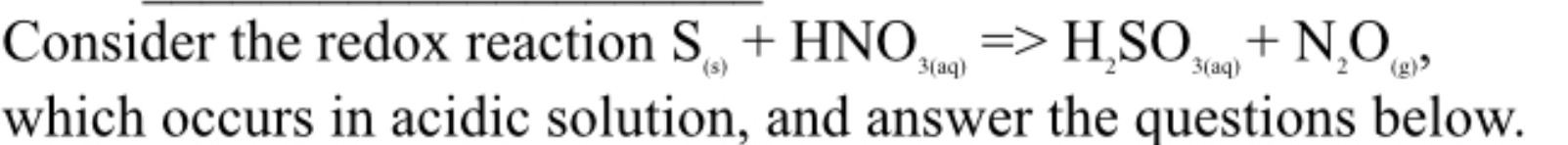# HSO NO,(g)>Consider the redox reaction SHNO,(s)3(аq)3(аq)which occurs in acidic solution, and answer the questions below

Question
26 views

What is the properly balanced reaction?help_outlineImage TranscriptioncloseHSO NO, (g)> Consider the redox reaction S HNO, (s) 3(аq) 3(аq) which occurs in acidic solution, and answer the questions below fullscreen
check_circle

Step 1

The oxidation number of the sulfur atom in S (s) and nitrogen atom in HNO3 are 0 and +5 respectively. Also, the oxidation number of the sulfur atom in H2SO3 and nitrogen atom in N2O is +4 and +1 respectively.

Step 2

In equation (1), the skeleton equation for the given reaction is represented and all the oxidation states are represented above the ionic species.

Step 3

The oxidation half for the given reac...

### Want to see the full answer?

See Solution

#### Want to see this answer and more?

Solutions are written by subject experts who are available 24/7. Questions are typically answered within 1 hour.*

See Solution
*Response times may vary by subject and question.
Tagged in

### Redox Reactions